# RD Sharma Solutions for Class 6 Chapter 7: Decimals Exercise 7.2

In this exercise, the students will learn that the decimals are another name of fractions whose denominators are 10, 100, 1000, etc. It mainly has problems based on determining the tenths place in the given set of decimals. RD Sharma Solutions for class 6 has problems based on CBSE textbook which are designed by subject matter experts. The students can refer RD Sharma Solutions for Class 6 Maths Chapter 7 Decimals Exercise 7.2 PDF which are provided here.

## RD Sharma Solutions for Class 6 Chapter 7: Decimals Exercise 7.2 Download PDF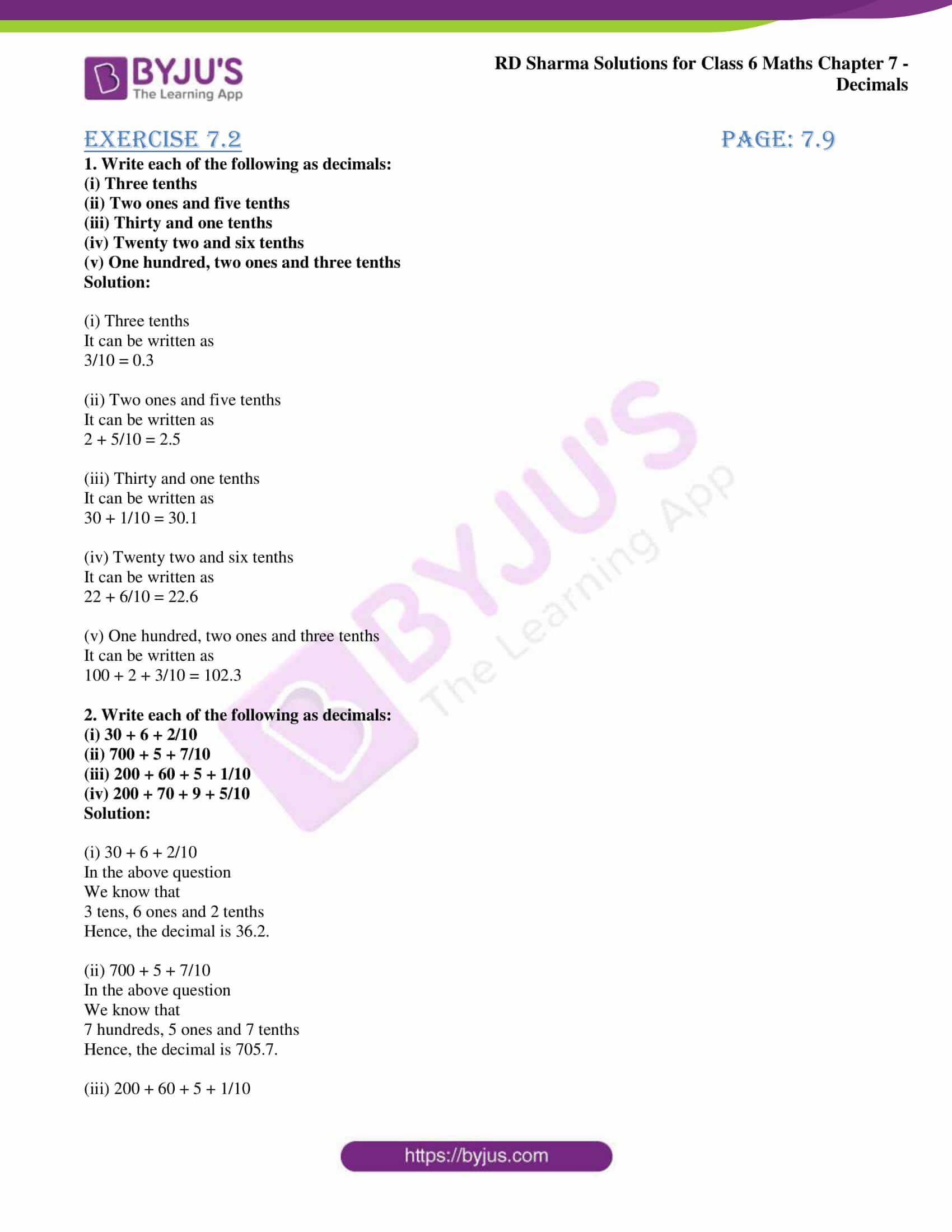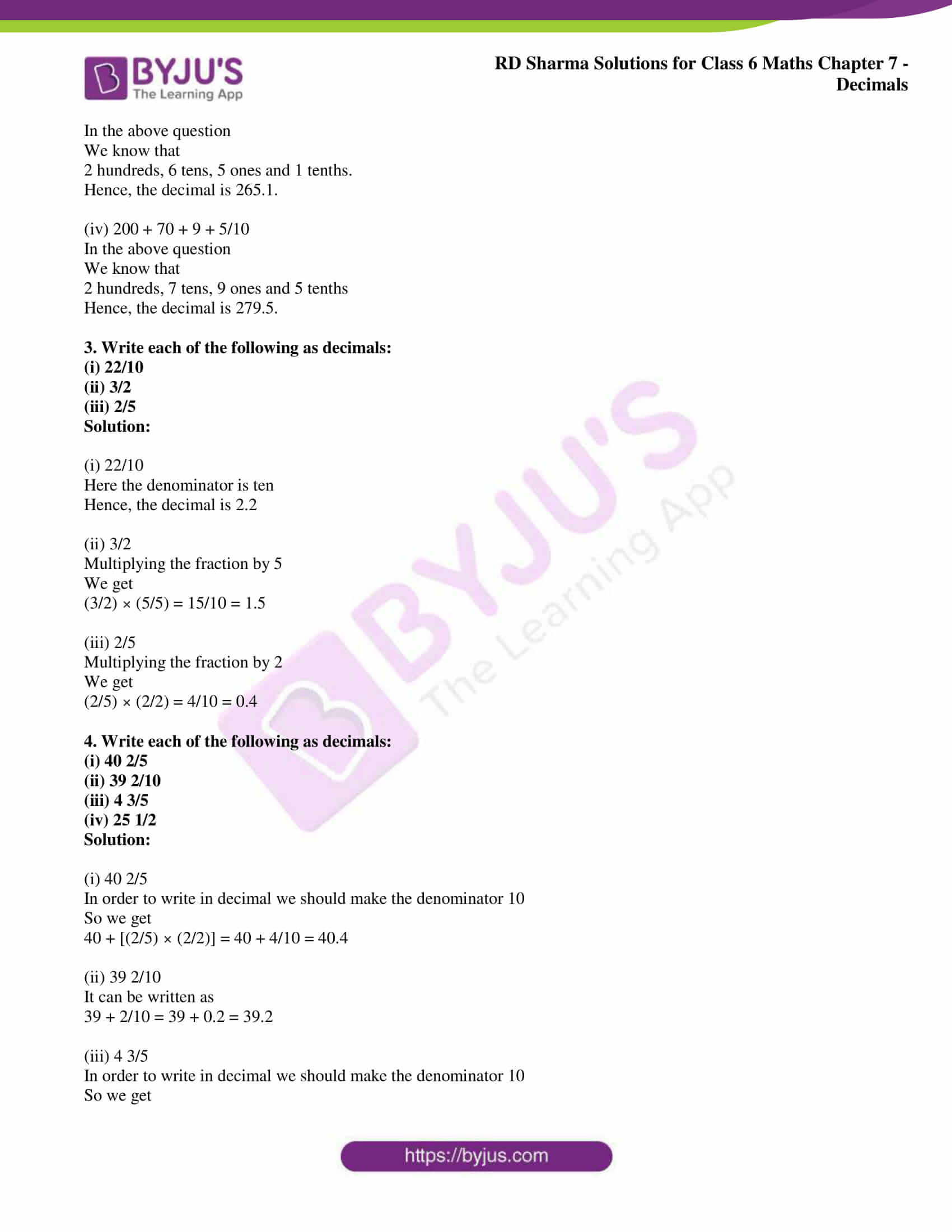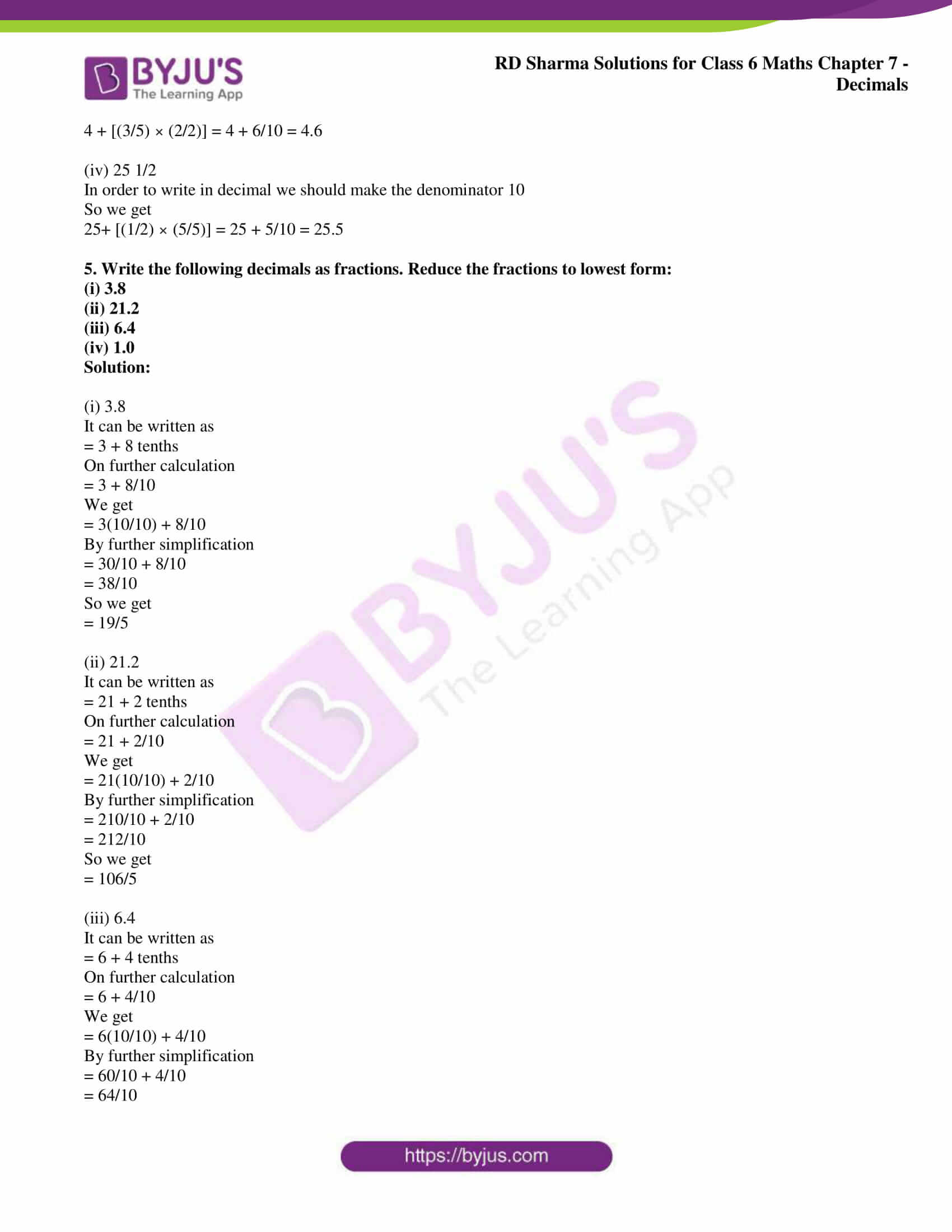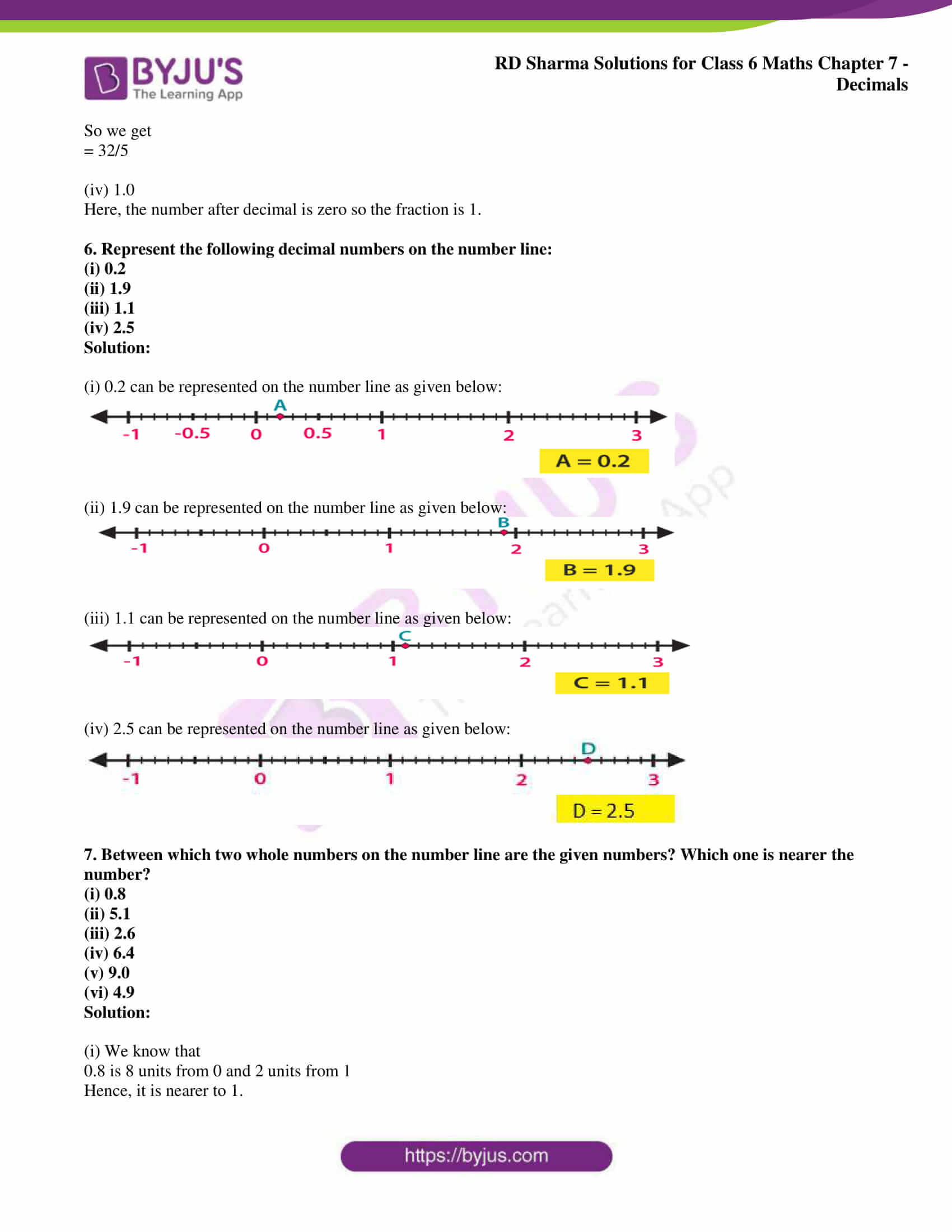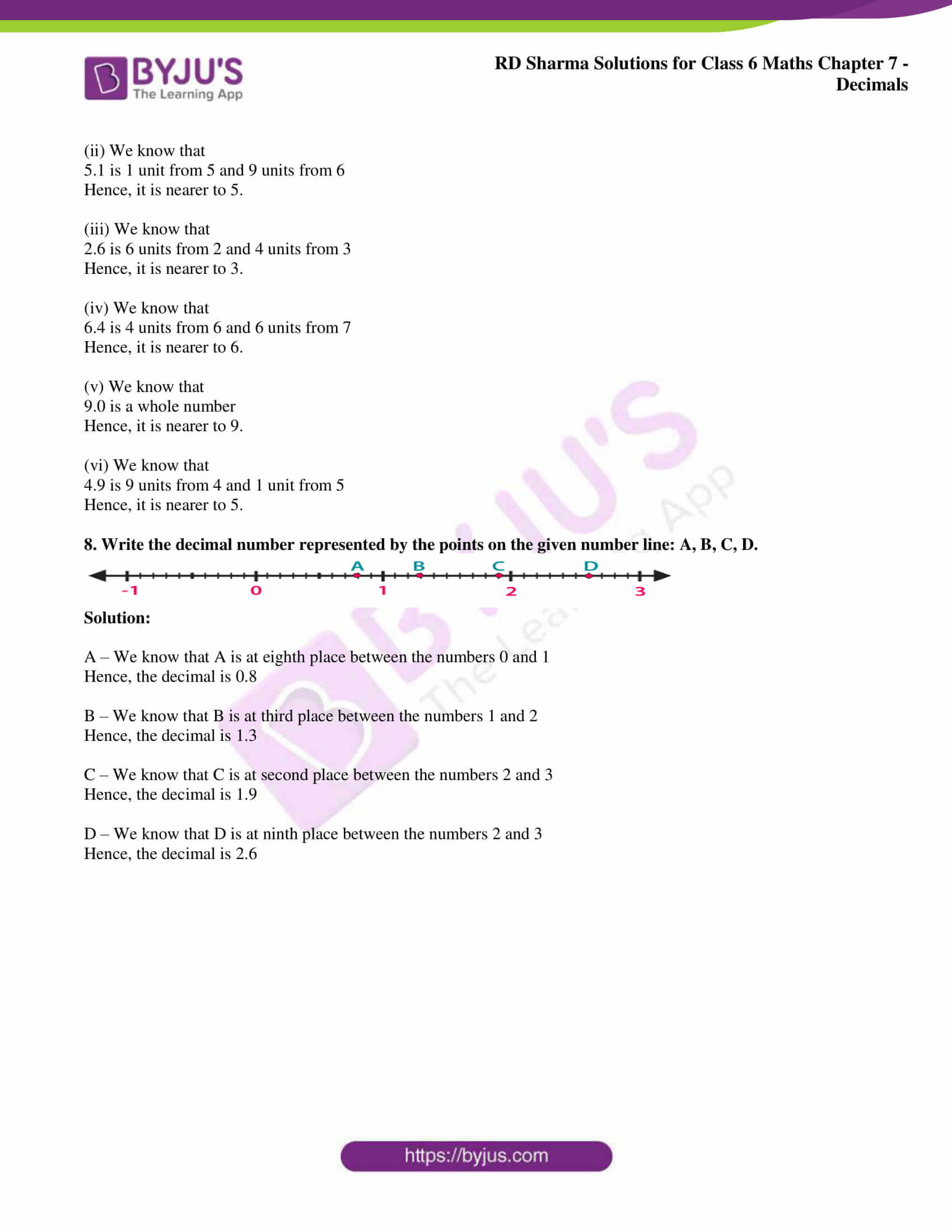### Access RD Sharma Solutions for Class 6 Chapter 7: Decimals Exercise 7.2

1. Write each of the following as decimals:

(i) Three tenths

(ii) Two ones and five tenths

(iii) Thirty and one tenths

(iv) Twenty two and six tenths

(v) One hundred, two ones and three tenths

Solution:

(i) Three tenths

It can be written as

3/10 = 0.3

(ii) Two ones and five tenths

It can be written as

2 + 5/10 = 2.5

(iii) Thirty and one tenths

It can be written as

30 + 1/10 = 30.1

(iv) Twenty two and six tenths

It can be written as

22 + 6/10 = 22.6

(v) One hundred, two ones and three tenths

It can be written as

100 + 2 + 3/10 = 102.3

2. Write each of the following as decimals:

(i) 30 + 6 + 2/10

(ii) 700 + 5 + 7/10

(iii) 200 + 60 + 5 + 1/10

(iv) 200 + 70 + 9 + 5/10

Solution:

(i) 30 + 6 + 2/10

In the above question

We know that

3 tens, 6 ones and 2 tenths

Hence, the decimal is 36.2.

(ii) 700 + 5 + 7/10

In the above question

We know that

7 hundreds, 5 ones and 7 tenths

Hence, the decimal is 705.7.

(iii) 200 + 60 + 5 + 1/10

In the above question

We know that

2 hundreds, 6 tens, 5 ones and 1 tenths.

Hence, the decimal is 265.1.

(iv) 200 + 70 + 9 + 5/10

In the above question

We know that

2 hundreds, 7 tens, 9 ones and 5 tenths

Hence, the decimal is 279.5.

3. Write each of the following as decimals:

(i) 22/10

(ii) 3/2

(iii) 2/5

Solution:

(i) 22/10

Here the denominator is ten

Hence, the decimal is 2.2

(ii) 3/2

Multiplying the fraction by 5

We get

(3/2) × (5/5) = 15/10 = 1.5

(iii) 2/5

Multiplying the fraction by 2

We get

(2/5) × (2/2) = 4/10 = 0.4

4. Write each of the following as decimals:

(i) 40 2/5

(ii) 39 2/10

(iii) 4 3/5

(iv) 25 1/2

Solution:

(i) 40 2/5

In order to write in decimal we should make the denominator 10

So we get

40 + [(2/5) × (2/2)] = 40 + 4/10 = 40.4

(ii) 39 2/10

It can be written as

39 + 2/10 = 39 + 0.2 = 39.2

(iii) 4 3/5

In order to write in decimal we should make the denominator 10

So we get

4 + [(3/5) × (2/2)] = 4 + 6/10 = 4.6

(iv) 25 1/2

In order to write in decimal we should make the denominator 10

So we get

25+ [(1/2) × (5/5)] = 25 + 5/10 = 25.5

5. Write the following decimals as fractions. Reduce the fractions to lowest form:

(i) 3.8

(ii) 21.2

(iii) 6.4

(iv) 1.0

Solution:

(i) 3.8

It can be written as

= 3 + 8 tenths

On further calculation

= 3 + 8/10

We get

= 3(10/10) + 8/10

By further simplification

= 30/10 + 8/10

= 38/10

So we get

= 19/5

(ii) 21.2

It can be written as

= 21 + 2 tenths

On further calculation

= 21 + 2/10

We get

= 21(10/10) + 2/10

By further simplification

= 210/10 + 2/10

= 212/10

So we get

= 106/5

(iii) 6.4

It can be written as

= 6 + 4 tenths

On further calculation

= 6 + 4/10

We get

= 6(10/10) + 4/10

By further simplification

= 60/10 + 4/10

= 64/10

So we get

= 32/5

(iv) 1.0

Here, the number after decimal is zero so the fraction is 1.

6. Represent the following decimal numbers on the number line:

(i) 0.2

(ii) 1.9

(iii) 1.1

(iv) 2.5

Solution:

(i) 0.2 can be represented on the number line as given below: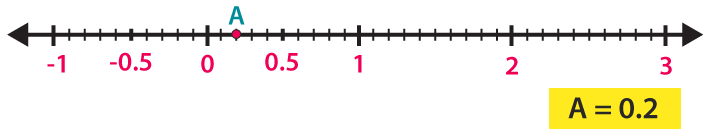(ii) 1.9 can be represented on the number line as given below: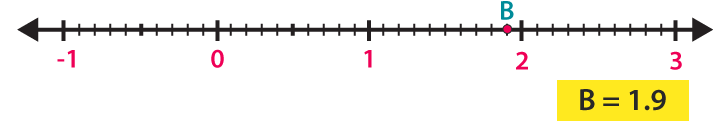(iii) 1.1 can be represented on the number line as given below: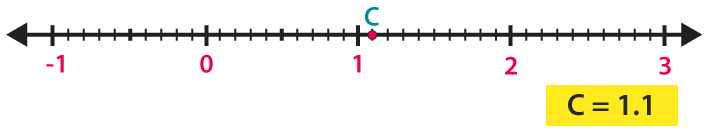(iv) 2.5 can be represented on the number line as given below: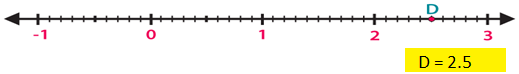7. Between which two whole numbers on the number line are the given numbers? Which one is nearer the number?

(i) 0.8

(ii) 5.1

(iii) 2.6

(iv) 6.4

(v) 9.0

(vi) 4.9

Solution:

(i) We know that

0.8 is 8 units from 0 and 2 units from 1

Hence, it is nearer to 1.

(ii) We know that

5.1 is 1 unit from 5 and 9 units from 6

Hence, it is nearer to 5.

(iii) We know that

2.6 is 6 units from 2 and 4 units from 3

Hence, it is nearer to 3.

(iv) We know that

6.4 is 4 units from 6 and 6 units from 7

Hence, it is nearer to 6.

(v) We know that

9.0 is a whole number

Hence, it is nearer to 9.

(vi) We know that

4.9 is 9 units from 4 and 1 unit from 5

Hence, it is nearer to 5.

8. Write the decimal number represented by the points on the given number line: A, B, C, D.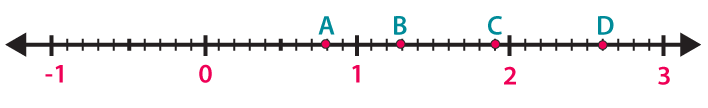Solution:

A – We know that A is at eighth place between the numbers 0 and 1

Hence, the decimal is 0.8

B – We know that B is at third place between the numbers 1 and 2

Hence, the decimal is 1.3

C – We know that C is at second place between the numbers 2 and 3

Hence, the decimal is 1.9

D – We know that D is at ninth place between the numbers 2 and 3

Hence, the decimal is 2.6